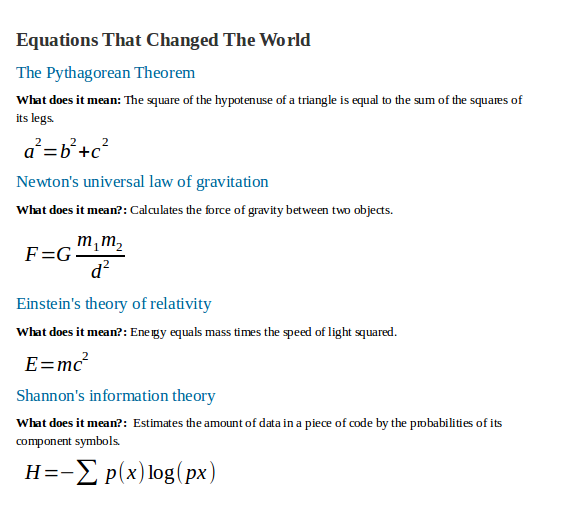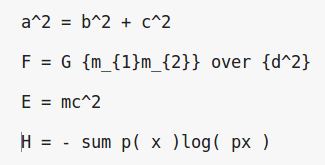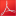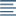## Math Exercise

Practice your skills in typing formulas using Math component of LibreOffice. In this exercise you will format a given text and type 4 formulas. The end result will look similar to the picture below.### Instructions

Download and open the file math-exercise-start.odt. You will work on this file.

Insert, type and format the equations. To help you type the equations you are given the exact format for each one.Change the Font Size for each Equation to Base Size: 16

Format the text in the document. Apply Heading 1 style in the title and Heading 2 for the title of each equation. Change font color in Heading 2. Your formatting does not have to look exactly like the solution.

•math-exercise-solution.pdf
•math-exercise-start.odt